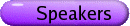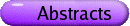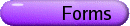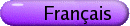L-Functions and Algebraic Curves Org: Yu-Ru Liu, David McKinnon and Michael Rubinstein (Waterloo)[PDF] AMIR AKBARY, Department of Mathematics and Computer Science, University of Lethbridge, 4401 University Drive West, Lethbridge, AB T1K 3M4 Mean Values of Product of Modular L-Functions [PDF] Let f be a newform of even weight k, level M and character y and let g be a newform of even weight l, level N and character h. We give a generalization of a theorem of Elliott, regarding the average values of Dirichlet L-functions, in the context of twisted product L-functions Lf,c(s0)[`(Lg,c(s0))] associated to f and g and Dirichlet characters c. IMIN CHEN, Simon Fraser University Diophantine equations via Galois representations [PDF] I will describe recent work on applying the method of Galois representations and modular forms to further cases of the generalized Fermat equation. ALINA COJOCARU, Princeton University, Dept. Mathematics, Fine Hall, Washington Road, Princeton, NJ 08544, USA Uniform results for Serre's Theorem for elliptic curves [PDF] Let E be an elliptic curve defined over Q. For a rational prime q, let rq be the mod q Galois representation of E. A classical result of Serre, proven in 1972, asserts that if E is without complex multiplication, then there exists a constant C(E) > 0 such that rq is surjective for any q > C(E). Serre asked whether the constant C(E) is absolute (i.e., it does not depend on E). In my presentation I will discuss function field and one-parameter average analogues of Serre's question. This is joint work with Chris Hall (Univ. of Texas at Austin). BRIAN CONREY, AIM JOHN FRIEDLANDER, University of Toronto Exceptional characters and the distribution of primes [PDF] We study some of the extremely strong statements about the distribution of prime numbers which follow from the (admittedly unlikely) assumption of the existence of exceptional Dirichlet characters. This is joint work with Henryk Iwaniec. ALEX GHITZA, McGill University A numerical exploration of mod p Hecke eigensystems [PDF] In his recent preprint on Serre's conjecture in level 1, Khare asks about the number of mod p Galois representations unramified outside p, or equivalently (thanks to his work), the number of level 1 mod p Hecke eigensystems. We report on some computational work on this problem and its generalizations. STEVE GONEK, University of Rochester, Rochester, NY 14627 A Statistical Model for the Riemann Zeta Function [PDF] The recent random matrix characteristic polynomial models for the Riemann zeta function and other L-functions have allowed us to predict answers to a variety of questions previously considered intractable. However, random matrices carry no arithmetical information, so one generally has to insert this component of the answer in an ad hoc manner. I will present a new model developed with C. Hughes and J. Keating that avoids this problem and illustrate its applications. HARALD HELFGOTT, Université de Montréal Growth and generation in SL2(Z/p) [PDF] Some groups can be generated slowly. Some groups can't. In some groups, sets can grow slowly. In some other groups, sets can't. What goes wrong when additive combinatorics goes non-abelian-and how can this "wrongness" help us where automorphic forms have not? ERNST KANI, Queen's University L-functions of certain quotient varieties [PDF] In this talk I plan to discuss the Hasse-Weil Zeta-function of (singular) quotient varieties Y = X/G, where G is a finite group acting on a smooth variety X/Q. Of particular interest here is the case that X is a modular product surface of level N, i.e., X = X(N) ×X(N), where X(N)/Q is the modular curve of level N. SHINYA KOYAMA, Zeta Institute The double Riemann zeta function [PDF] The double Riemann zeta function (zÄz)(s) is defined by a double Euler product over pairs of primes (p,q). Any nontrivial zero r of (zÄz)(s) is given by a sum of zeros of z(s). Namely, there exists a pair of zeros r1 and r2 such that r = r1+r2. The aim of this talk is to introduce a basic theory of the double Riemann zeta function, and discuss its possible applications. The first possibility is to enlarge the zero-free region of z(s). We obtain an explicit form of the (p,q)-Euler factors and show that the double Euler product is absolutely convergent in Â(s) > 2. Conjecturally it should be convergent in Â(s) > 3/2, which implies that z(s) is zero-free in Â(s) > 3/4. Thus any improvement of our current result would give a new result toward the RH. The second application is to improve the ratio N0(T)/N(T). Since a zero r = r1+r2 is simple only if both r1 and r2 are simple, an estimate of the ratio of simple zeros for (zÄz)(s) can possibly improve the ratio N0(T)/N(T) for z(s). JUNG-JO LEE, Queen's University Dirichlet Series and Hyperelliptic Curves, Part 2 [PDF] We will continue our investigation of attaching Dirichlet series to hyperelliptic and superelliptic curves and deduce various arithmetic results from analytic properties of these series. This is joint work with M. Ram Murty. ADAM LOGAN, University of Liverpool (England) Descent by Richelot isogeny on the Jacobians of plane quartics [PDF] We discuss theoretical and practical aspects of Richelot isogenies on the Jacobians of curves of genus 3, including their application to calculating invariants of the curves. GREG MARTIN, University of British Columbia Inequities in the Shanks-Rényi Prime Number Race [PDF] Let p(x;q,a) denote the number of primes up to x that are congruent to a modulo q. Inequalities of the type p(x;q,a) > p(x;q,b) are more likely to hold if a is a non-square modulo q and b is a square modulo q (the so-called "Chebyshev Bias" in comparative prime number theory).  However, the tendencies of the various p(x;q,a) (for nonsquares a) to dominate p(x;q,b) have different strengths.  A related phenomenon is that the six possible inequalities of the form  p(x;q,a1) >  p(x;q,a2) >  p(x;q,a3), with a1, a2, a3 all non-squares modulo q, are not equally likely; some orderings are preferred over others.  For given values q,a,b,..., these tendencies can be quantified and computed, but only using laborious numerical integration of functions involving zeros of the appropriate Dirichlet L-functions.  In this talk we describe a framework for explaining which nonsquares a are most dominant for a given square b, for example, based only on elementary properties of the congruence classes a modulo q rather than the complicated computations just mentioned. These elementary properties, on the other hand, do derive from consideration of differences in the distributions of zeros of various L-functions, for example those corresponding to odd and even characters. KUMAR MURTY, University of Toronto, 100 St. George Street, Toronto, ON M5S 3G3 On the order of vanishing of L-functions at the central critical point [PDF] We formulate a pair correlation hypothesis for certain general L-functions and discuss the implication of such a hypothesis for the order of vanishing at the central critical point. DOUG ULMER, University of Arizona Abelian varieties of large analytic rank over function fields [PDF] I will discuss a simple linear algebra fact which allows one to produce lots of L-functions with large order zeroes at the critical point. Two sample applications: (1) for every p and every g there exist geometrically simple, non-isotrivial abelian varieties of dimension g over Fp(t) with arbitrarily large analytic rank; and (2) if E is any elliptic curve over Fq(t) with j-invariant not in Fq, then E obtains arbitrarily large analytic rank over extensions of the form Fq(u), where t is a rational function of u.